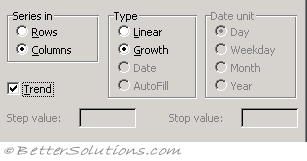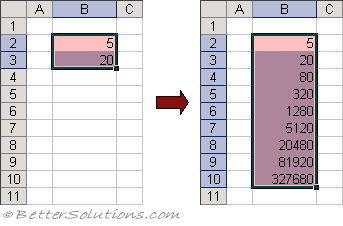# Growth Trends

Multiplies the starting value by each value in turn by the step value
Each cell is multiplied by the "step" value.Growth - Creates a growth series or geometric growth trend. If the Trend box is cleared, a series is calculated by multiplying the value in the Step value box by each cell value in turn. If the Trend box is selected, the value in the Step value box is ignored, and a geometric growth trend is calculated based on the selected values. This is covered in more detail on a subsequent page.
Trend - Calculates a geometric growth trend using the values that appear at the top or to the left of the selection. Any value in the Step value box is ignored if the Trend check box is selected. Excel will calculate the average change between the values already entered and uses that information to calculate a series to populate the remaining cells.